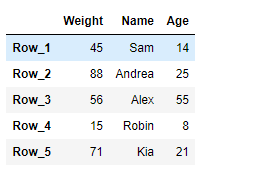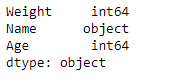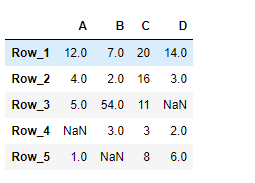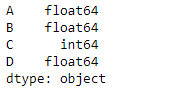Related Articles
Python | Pandas DataFrame.dtypes
• Difficulty Level : Basic
• Last Updated : 20 Feb, 2019

Pandas DataFrame is a two-dimensional size-mutable, potentially heterogeneous tabular data structure with labeled axes (rows and columns). Arithmetic operations align on both row and column labels. It can be thought of as a dict-like container for Series objects. This is the primary data structure of the Pandas.

Pandas` DataFrame.dtypes` attribute return the dtypes in the DataFrame. It returns a Series with the data type of each column.

Syntax: DataFrame.dtypes

Parameter : None

Returns : dtype of each column

Example #1: Use `DataFrame.dtypes` attribute to find out the data type (dtype) of each column in the given dataframe.

 `# importing pandas as pd``import` `pandas as pd`` ` `# Creating the DataFrame``df ``=` `pd.DataFrame({``'Weight'``:[``45``, ``88``, ``56``, ``15``, ``71``],``                   ``'Name'``:[``'Sam'``, ``'Andrea'``, ``'Alex'``, ``'Robin'``, ``'Kia'``],``                   ``'Age'``:[``14``, ``25``, ``55``, ``8``, ``21``]})`` ` `# Create the index``index_ ``=` `[``'Row_1'``, ``'Row_2'``, ``'Row_3'``, ``'Row_4'``, ``'Row_5'``]`` ` `# Set the index``df.index ``=` `index_`` ` `# Print the DataFrame``print``(df)`

Output :Now we will use `DataFrame.dtypes` attribute to find out the data type of each column in the given dataframe.

 `# return the dtype of each column``result ``=` `df.dtypes`` ` `# Print the result``print``(result)`

Output :As we can see in the output, the `DataFrame.dtypes` attribute has successfully returned the data types of each column in the given dataframe.

Example #2: Use `DataFrame.dtypes` attribute to find out the data type (dtype) of each column in the given dataframe.

 `# importing pandas as pd``import` `pandas as pd`` ` `# Creating the DataFrame``df ``=` `pd.DataFrame({``"A"``:[``12``, ``4``, ``5``, ``None``, ``1``], ``                   ``"B"``:[``7``, ``2``, ``54``, ``3``, ``None``], ``                   ``"C"``:[``20``, ``16``, ``11``, ``3``, ``8``], ``                   ``"D"``:[``14``, ``3``, ``None``, ``2``, ``6``]}) `` ` `# Create the index``index_ ``=` `[``'Row_1'``, ``'Row_2'``, ``'Row_3'``, ``'Row_4'``, ``'Row_5'``]`` ` `# Set the index``df.index ``=` `index_`` ` `# Print the DataFrame``print``(df)`

Output :Now we will use `DataFrame.dtypes` attribute to find out the data type of each column in the given dataframe.

 `# return the dtype of each column``result ``=` `df.dtypes`` ` `# Print the result``print``(result)`

Output :As we can see in the output, the `DataFrame.dtypes` attribute has successfully returned the data types of each column in the given dataframe.

Attention geek! Strengthen your foundations with the Python Programming Foundation Course and learn the basics.

To begin with, your interview preparations Enhance your Data Structures concepts with the Python DS Course. And to begin with your Machine Learning Journey, join the Machine Learning – Basic Level Course

My Personal Notes arrow_drop_up